# DFIT

## Brief

DFIT is the diagnostic fracture injection test or the fracture calibration injection falloff test performed to estimate ISIP, fracture closure pressure, fluid efficiency, leak off coefficient, formation permeability and pressure before the main hydraulic fracture treatment.

## Math & Physics

in progress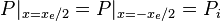$P |_{x=x_e/2} = P |_{x=-x_e/2} = P_i$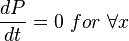$\frac{dP}{dt} =0\ for \ \forall x$

From Darcy's law: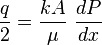$\frac{q}{2}=\frac{kA}{\mu}\ \frac{dP}{dx}$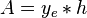$A =y_e*h$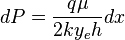$dP=\frac{q \mu}{2ky_eh} dx$

Integration gives: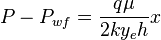$P-P_{wf}=\frac{q \mu}{2ky_eh} x$

Since average pressure is: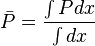$\bar P = \frac{\int P dx}{\int dx}$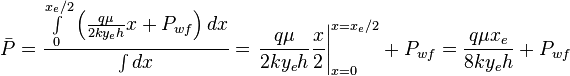$\bar P = \frac{ \int \limits_{0}^{x_e/2} \left ( \frac{q \mu}{2ky_eh} x + P_{wf} \right ) dx}{\int dx} = \left. \frac{q \mu}{2ky_eh} \frac{x}{2} \right|_{x=0}^{x=x_e/2} + P_{wf} = \frac{q \mu x_e}{8ky_eh} + P_{wf}$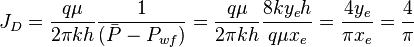$J_D=\frac{q \mu}{2 \pi k h} \frac{1}{( \bar P - P_{wf})} =\frac{q \mu}{2 \pi k h} \frac{8ky_eh}{q \mu x_e} = \frac{4y_e}{\pi x_e}=\frac{4}{\pi}$

## Nomenclature$A$ = cross-sectional area, cm2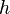$h$ = thickness, m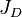$J_D$ = dimensionless productivity index, dimensionless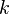$k$ = permeability, d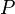$P$ = pressure, atm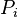$P_i$ = initial pressure, atm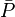$\bar P$ = average pressure, atm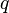$q$ = flow rate, cm3/sec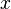$x$ = length, m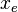$x_e$ = drinage area length, m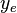$y_e$ = drinage area width, m

### Greek symbols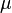$\mu$ =viscosity, cp